# Equations Worksheet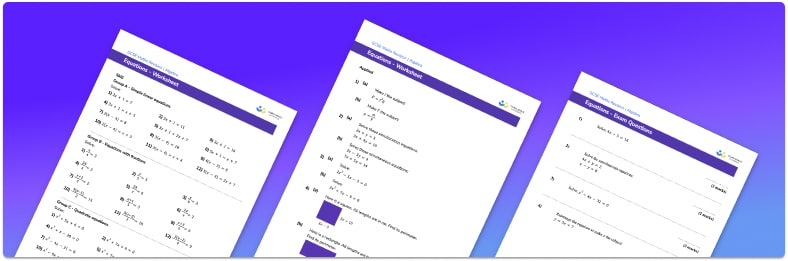• This field is for validation purposes and should be left unchanged.

You can unsubscribe at any time (each email we send will contain an easy way to unsubscribe). To find out more about how we use your data, see our privacy policy.

• Section 1 of the linear equations worksheet contains 36 skills-based questions, in 3 groups to support differentiation
• Section 2 contains 4 applied linear equations questions with a mix of worded problems and deeper problem solving questions
• Section 3 contains 4 foundation and higher level GCSE exam questions
• Answers and a mark scheme for all linear equations questions
• Follows variation theory with plenty of opportunities for students to work independently at their own level
• All questions created by fully qualified expert secondary maths teachers
• Suitable for GCSE maths revision for AQA, OCR and Edexcel exam boards

### Linear equations at a glance

An equation is an algebraic expression that is equal to something. We can solve different types of equations to work out the value of an unknown variable. Solving equations uses many of the same skills as rearranging formulae.

Solving linear equations involves applying an inverse operation to both sides of the equation. There are different types of linear equations we can solve including one-step equations, two-step equations and multi-step equations. Solving linear equations can involve expanding brackets in an expression and having unknown variables on both sides of the equals sign.

Solving quadratic equations involves using a particular method to calculate the value of the unknown variable that includes a squared term.

One method to solve a quadratic equation is to set the quadratic expression equal to zero and factorise into brackets. A second method to solve a quadratic equation is to use the quadratic formula. To do this we use the coefficients of the variables which can be integers, decimals or fractions, and substitute them into the quadratic formula. A strong understanding of substitution and the order of operations is important for this. Another method to solve a quadratic equation is to use the completing the square method. This method required a strong understanding of squares and square roots.

Equations also involve solving simultaneous equations. Here there are two equations and two unknowns which need to be found. One way to do this is to use the elimination method and often requires one or both equations to be multiplied by an integer before the addition or subtraction of the equations to eliminate one of the variables. A second method is the substitution method, where one equation is substituted into the other equation. The substitution method is more often used to solve simultaneous equations where one equation is a linear equation and one is non-linear.

Equations may also involve algebraic fractions which require several different methods and skills to simplify and solve.

Looking forward, students can then progress to additional algebra worksheets, for example a simplifying expressions worksheet or inequalities worksheet.For more teaching and learning support on Algebra our GCSE maths lessons provide step by step support for all GCSE maths concepts.

## Related worksheets

Solving Equations with Fractions Worksheet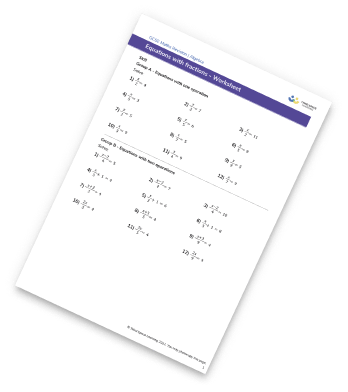Completing The Square Worksheet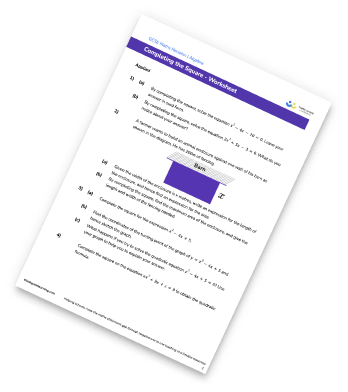Linear Equations Worksheet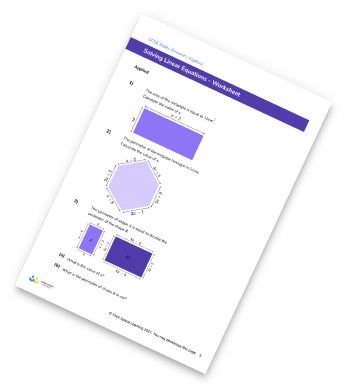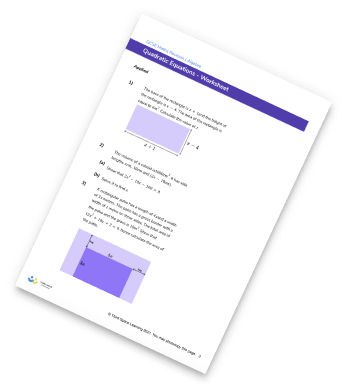## Do you have KS4 students who need more focused attention to succeed at GCSE?There will be students in your class who require individual attention to help them succeed in their maths GCSEs. In a class of 30, it’s not always easy to provide.

Help your students feel confident with exam-style questions and the strategies they’ll need to answer them correctly with our dedicated GCSE maths revision programme.

Lessons are selected to provide support where each student needs it most, and specially-trained GCSE maths tutors adapt the pitch and pace of each lesson. This ensures a personalised revision programme that raises grades and boosts confidence.

Find out more